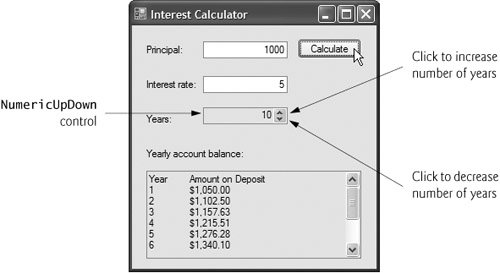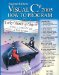# NumericUpDown Control

At times, we will want to restrict a user's input choices to a specific range of numeric values. This is the purpose of the NumericUpDown control. This control appears as a TextBox, with two small Buttons on the right sideone with an up arrow and one with a down arrow. By default, a user can type numeric values into this control as if it were a TextBox or click the up and down arrows to increase or decrease the value in the control, respectively. The largest and smallest values in the range are specified with the Maximum and Minimum properties, respectively (both of type decimal). The Increment property (also of type decimal) specifies by how much the current number in the control changes when the user clicks the control's up and down arrows. Figure 13.35 describes common properties and a common event of class NumericUpDown.

Figure 13.35. NumericUpDown properties and event.

NumericUpDown properties and event

Description

Common Properties

Increment

Specifies by how much the current number in the control changes when the user clicks the control's up and down arrows.

Maximum

Largest value in the control's range.

Minimum

Smallest value in the control's range.

UpDownAlign

Modifies the alignment of the up and down Buttons on the NumericUpDown control. This property can be used to display these Buttons either to the left or to the right of the control.

Value

The numeric value currently displayed in the control.

Common Event

ValueChanged

This event is raised when the value in the control is changed. This is the default event for the NumericUpDown control.

Figure 13.36 demonstrates using a NumericUpDown control for a GUI that calculates interest rate. The calculations performed in this application are similar to those performed in Fig. 6.6. TextBoxes are used to input the principal and interest rate amounts, and a NumericUpDown control is used to input the number of years for which we want to calculate interest.

Figure 13.36. Demonstrating the NumericUpDown control.

(This item is displayed on pages 631 - 632 in the print version)

 ``` 1 // Fig. 13.36: interestCalculatorForm.cs 2 // Demonstrating the NumericUpDown control. 3 using System; 4 using System.Windows.Forms; 5 6 public partial class interestCalculatorForm : Form 7 { ``` 8 // default constructor 9 public interestCalculatorForm() 10 { 11 InitializeComponent(); 12 } // end constructor 13 14 private void calculateButton_Click( 15 object sender, EventArgs e ) 16 { 17 // declare variables to store user input 18 decimal principal; // store principal 19 double rate; // store interest rate 20 int year; // store number of years 21 decimal amount; // store amount 22 string output; // store output 23 24 // retrieve user input 25 principal = Convert.ToDecimal( principalTextBox.Text ); 26 rate = Convert.ToDouble( interestTextBox.Text ); 27 year = Convert.ToInt32( yearUpDown.Value ); 28 29 // set output header 30 output = "Year Amount on Deposit "; 31 32 // calculate amount after each year and append to output 33 for ( int yearCounter = 1; yearCounter <= year; yearCounter++ ) 34 { 35 amount = principal * 36 ( ( decimal ) Math.Pow( ( 1 + rate / 100 ), yearCounter ) ); 37 output += ( yearCounter + " " + 38 string.Format( "{0:C}", amount ) + " " ); 39 } // end for 40 41 displayTextBox.Text = output; // display result 42 } // end method calculateButton_Click 43 } // end class interestCalculatorFormFor the NumericUpDown control named yearUpDown, we set the Minimum property to 1 and the Maximum property to 10. We left the Increment property set to 1, its default value. These settings specify that users can enter a number of years in the range 1 to 10 in increments of 1. If we had set the Increment to 0.5, we could also input values such as 1.5 or 2.5. We set the NumericUpDown's ReadOnly property to true to indicate that the user can cannot type a number into the control to make a selection. Thus, the user must click the up and down arrows to modify the value in the control. By default, the ReadOnly property is set to false. The output for this application is displayed in a multiline read-only TextBox with a vertical scrollbar, so the user can scroll through the entire output.Visual C# 2005 How to Program (2nd Edition)
ISBN: 0131525239
EAN: 2147483647
Year: 2004
Pages: 600

Similar book on Amazon